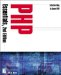# Variables

You create variables to represent data. For instance, the following variable holds a value for sales tax:

` \$sales_tax = 0.0875; `

This variable holds a SQL statement:

` \$sql = "SELECT * FROM MY_TABLE"; `

You can refer to the value of other variables when determining the value of a new variable:

` \$tax_total = \$sales_tax * \$sub_total; `

The following are true of variable names:

• They begin with a dollar sign (\$).

• They cannot begin with a numeric character.

• They can contain numbers and the underscore character (_).

• They are case-sensitive.

Here are some common variable types:

• floats

• integers

• strings

These types are determined by PHP, based on the context in which they appear.

### Floats

Each of the following variables is a float, or floating-point number. Floats are also known as "numbers with decimal points."

` \$a = 1.552; \$b = 0.964; \$sales_tax = 0.875; `

### Integers

Integers are positive or negative whole numbers, zero, or "numbers without decimal points." Each of the following variables is an integer.

` \$a = 15; \$b = -521; `

### Strings

A series of characters grouped within double quotes is considered a string:

` \$a - "I am a string."; \$b = "<P>This book is <strong>cool</strong>!"; `

You can also reference other variables within your string, which will be replaced when your script is executed. For example:

` \$num = 57; // an integer \$my_string = "I read this book \$num times!"; // a string `

When you run the script, \$my_string will become "I read this book 57 times!"

### Variables from HTML Forms

Depending on the method of your HTML form (GET or POST), the variables will be part of the \$_POST or \$_GET superglobal associative array. The name of the input field will become the name of the variable. For example, when a form is sent using the POST method, the following input field produces the variable \$_POST[first_name]:

` <input type="text" name="first_name" size="20"> `

If the method of this form were GET, this variable would be \$_GET[first_name].

Like variables from forms, variables from cookies are kept in a superglobal associative array called \$_COOKIE. If you set a cookie called user with a value of Joe Smith, like so:

` SetCookie ("user", "Joe Smith", time()+3600); `

a variable called user is placed in \$_COOKIE, with a value of Joe Smith. You then refer to \$_COOKIE[user] to get that value.

### Environment Variables

When a Web browser makes a request of a Web server, it sends along with the request a list of extra variables called environment variables. They can be very useful for displaying dynamic content or authorizing users.

By default, environment variables are available to PHP scripts as \$VAR_NAME. However, to be absolutely sure that you're reading the correct value, you can use the getenv() function to assign a value to a variable of your choice. Following are some common environment variables:

REMOTE_ADDR gets the IP address of the machine making the request. For example:

` <?php           \$remote_address = getenv("REMOTE_ADDR");           echo "Your IP address is \$remote_address."; ?> `

HTTP_USER_AGENT gets the browser type, browser version, language encoding, and platform. For example:

` <?php           \$browser_type = getenv("HTTP_USER_AGENT");           echo "You are using \$browser_type."; ?> `

For a list of HTTP environment variables and their descriptions, visit http://hoohoo.ncsa.uiuc.edu/cgi/env.html.

### Arrays

Simply put, arrays are sets of variables that are contained as a group. In the following example, \$fave_colors is an array that contains strings representing array elements. In this case, the array elements (0 to 3) are names of colors.

` \$fave_colors = "red"; \$fave_colors = "blue"; \$fave_colors = "black"; \$fave_colors = "white"; `

Array elements are counted with 0 as the first position in the numerical index.PHP Essentials, 2nd Edition
ISBN: 1931841349
EAN: 2147483647
Year: 2002
Pages: 74